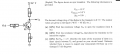# BJT Transistor finding Vc,Ic ..etc

#### beggi9

Joined Mar 3, 2016
42a) I got from Kirchoff that Vbb=0.7V

b) I assumed Bdc*Ib=Ic and that Ic=Ie. I then calculated Ic by using kirchoff for CE and got Ib=5.58*10^-3A
From there I got Ib=5.85*10^-5A so Vbb=8.89V

c) Here I'm really not certain, I put Vc=0V and therefore assumed Vcc must drop across the 1000ohm's (R1) so
12=1000*Ic ----> Ic = 1.2*10^-3
I don't know if I can assume Ie=1.2*10^-3A but if I do the voltage drop across R2 would be 1.2V.
So I would have 4V-40.000ohm*Ib-0.7V-1.2V=0
and therefor Ib=5.25*10^-5A.
So I would guess the transistor would be on but it wouldn't be on the linear phase, that is Ib*Bdc ≠ Ic

Last edited:

#### dl324

Joined Mar 30, 2015
15,511
a) I got from Kirchoff that Vbb=0.7V
Lets start here...

Are you required to use KVL? Have you accounted for all of the voltages in that loop?

#### dannyf

Joined Sep 13, 2015
2,197
From there I got Ib=5.85*10^-5A so Vbb=3.04V
Vbb = Ie * R2 + 0.7v + Ib * Rb > 0.7v+(12-0.3)/2 >> 3.04v.

#### beggi9

Joined Mar 3, 2016
42
Lets start here...

Are you required to use KVL? Have you accounted for all of the voltages in that loop?
No I'm not reaquired to use KVL. But hmm perhaps not, there would be a voltage drop over R2 as well right?

#### beggi9

Joined Mar 3, 2016
42
Vbb = Ie * R2 + 0.7v + Ib * Rb > 0.7v+(12-0.3)/2 >> 3.04v.
Wow yeah sorry about that, it should be 8.89V not 3.04V

#### dl324

Joined Mar 30, 2015
15,511
No I'm not reaquired to use KVL. But hmm perhaps not, there would be a voltage drop over R2 as well right?
IMO, this problem isn't a very good because it doesn't provide enough parameters.

What base-emitter voltage were you taught that a transistor starts to turn on at? 0.7 is generally considered to be the fully on voltage but, in actuality, it's usually larger.

When the transistor is just starting to turn on, there will be drops across Rb and R2.

•beggi9

#### WBahn

Joined Mar 31, 2012
28,173
I agree that the question is a bit ambiguous. It may or may not actually be depending on how you are presently modeling a transistor.

What have you been taught constitutes the boundary between a transistor being "on" and it being "off"?

This is often taken to be the state in which all of the voltage requirements for forward conduction are met but that the current is still zero -- it's right on the edge and any greater Vbe will have it conducting current while any less Vbe will move it into cutoff. If this is coupled with the common assumption that Vbe is a constant if the transistor is conducting leads to the answer you got. But it's important that you understand WHY this is the answer.

For part C, it is somewhat important to know what the heat dissipation capabilities of each component are. ANY component that is dissipating power will "heat up", but the question is almost certainly asking if any components will heat up "too much", which is a pretty vague criteria.

Before trying to answer that part of it, determine how much power each component will be dissipating. When looking at the transistor, keep in mind that the collector is clamped hard at 0 V.